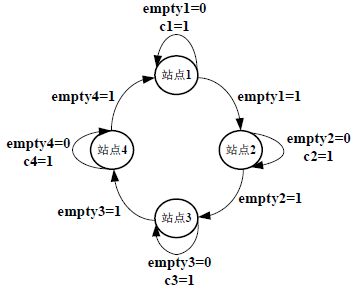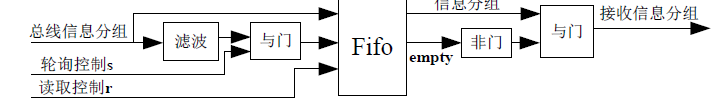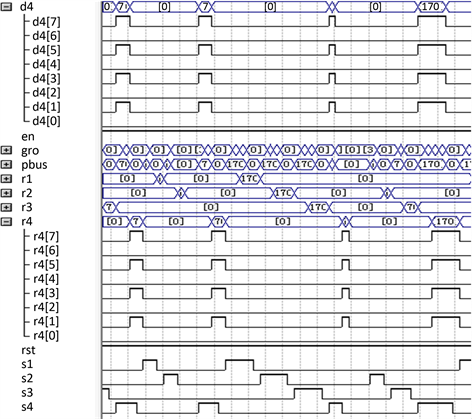# 基于完全服务轮询机制MAC协议的FPGA设计FPGA Design of MAC Protocol Based on Exhaustive Service Polling Mechanism

DOI: 10.12677/CSA.2018.85083, PDF, HTML, XML, 下载: 611  浏览: 956

Abstract: MAC protocol is a direct link between physical and network layer, which is one of the key protocols to ensure high efficient communication. According to the characteristics of polling system, this pa-per presents a FPGA design of MAC protocol based on exhaustive service polling mechanism. The design makes full use of the characteristics of flexibility and reconfigurability of FPGA. The method of combination of the hardware circuit description language Verilog HDL and the principle diagram is adopted, using Quartus II 8.0 to carry on the synthesis and routing, testing in the DE2. The design has the characteristics of good real-time, high reliability, strong portability, and can effectively reduce the transmission delay, and improve the bus utilization. The system designed can be widely used in wireless sensor networks, Ad Hoc networks, mobile communications networks, and net-working and other fields.

1. 引言

2. 系统原理

MAC (Multiple Access Control)协议，即多路访问控制协议，该协议决定了节点什么时候允许发送分组，而且通常控制对物理层的所有访问。共分为三大类：1) 按信道划分，将信道划分为较小的“段”，一般有频分多址(FDMA)、时分多址(TDMA)、码分多址(CDMA)。2) 随即访问，即不划分信道，允许碰撞发生并想办法从“碰撞”恢复。一般而言为竞争类的MAC协议，当然也有分配类和混合类的协议。对于WLAN，其中竞争类协议主要有CSMA，MACA，MACAW，FAMA，BTMA等。而非竞争类的协议主要有FPRP等协议。3) 轮询，主结点轮流“邀请”从属结点发送数据。

$\begin{array}{l}{G}_{i}\left({z}_{1},{z}_{2},\cdots ,{z}_{N}\right)=\underset{{x}_{1}=0}{\overset{\infty }{\sum }}\underset{{x}_{2}=0}{\overset{\infty }{\sum }}\cdots \underset{{x}_{i}=0}{\overset{\infty }{\sum }}\cdots \underset{{x}_{N}=0}{\overset{\infty }{\sum }}{\pi }_{i}\left({x}_{1},{x}_{2},\cdots ,{x}_{i},\cdots {x}_{N}\right)\\ {z}_{1}^{{x}_{1}}{z}_{2}^{{x}_{2}}\cdots {z}_{i}^{{x}_{i}}\cdots {z}_{N}^{{x}_{N}}\left(i=1,2,\cdots ,N\right)\end{array}$ (1)

$F\left({z}_{i}\right)=E\left[{z}_{i}^{{v}_{i},m}\right]=A\left(B\left({z}_{i}F\left({z}_{i}\right)\right)\right)\text{}\left(i=1,2,\cdots ,N\right)$

${t}_{n}+1$ 时刻开始对 $i+1$ 号站点服务，有关系式：

$\left\{\begin{array}{l}{\xi }_{j}\left(n+1\right)={\xi }_{j}\left(n\right)+{\eta }_{j}\left({v}_{i}\right)+{\mu }_{j}\left({u}_{i}\right)\\ {\xi }_{i}\left(n+1\right)={\mu }_{j}\left({u}_{i}\right)\end{array}\text{}\left(j\ne i\right)$ (2)

$\begin{array}{l}{G}_{i+1}\left({z}_{1},{z}_{2},\cdots ,{z}_{i},\cdots ,{z}_{N}\right)=\underset{n\to \infty }{\mathrm{lim}}E\left[\underset{j=1}{\overset{N}{\prod }}{z}_{j}^{{\xi }_{j}^{\left(n+1\right)}}\right]\\ =\underset{n\to \infty }{\mathrm{lim}}E\left[\underset{j}{\overset{N}{\prod }}{z}_{j}^{{\xi }_{j}\left(n\right)+{\mu }_{j}\left({u}_{i}\right)+{\eta }_{j}\left({v}_{i}\right)}\cdot {z}_{i}^{{\mu }_{j}\left({u}_{i}\right)}\right]=R\left[\underset{j=1}{\overset{N}{\prod }}{A}_{j}\left({z}_{j}\right)\right]\underset{n\to \infty }{\mathrm{lim}E}\left[\left(\underset{\begin{array}{l}j=1\\ j\ne 1\end{array}}{\overset{N}{\prod }}{z}_{j}^{{\xi }_{j}\left(n\right)}\right)\left(\underset{\begin{array}{l}j=1\\ j\ne 1\end{array}}{\overset{N}{\prod }}{z}_{j}^{{\eta }_{j}\left({v}_{i}\right)}\right)\right]\\ =R\left[\underset{j=1}{\overset{N}{\prod }}{A}_{j}\left({z}_{j}\right)\right]{G}_{i}\left[{z}_{1},{z}_{2},\cdots ,{z}_{i-1},{B}_{i}\left(\underset{\begin{array}{l}j=1\\ j\ne 1\end{array}}{\overset{N}{\prod }}{A}_{j}\left({z}_{j}\right)\right)\text{}F\left(\underset{\begin{array}{l}j=1\\ j\ne 1\end{array}}{\overset{N}{\prod }}{A}_{j}\left({z}_{j}\right)\right),{z}_{i+1},\cdot \cdot \cdot ,{z}_{N}\right]\text{}\left(i=1,2,\cdot \cdot \cdot ,N\right)\text{}\end{array}$ (3)

${g}_{i}\left(j\right)=\underset{{z}_{1},{z}_{2},\cdot \cdot \cdot ,{z}_{i},\cdot \cdot \cdot ,{z}_{N}\to 1}{\mathrm{lim}}\frac{\partial {G}_{i}\left({z}_{1},{z}_{2},\cdots ,{z}_{i}\cdots ,{z}_{N}\right)}{\partial {z}_{j}}\left(i=1,2,\cdot \cdot \cdot ,N;j=1,2,\cdot \cdot \cdot ,N\right)$

${g}_{i}\left(i\right)=\frac{N\gamma \lambda \left(1-\rho \right)}{1-N\rho }$ (4)

$\begin{array}{l}\stackrel{¯}{\theta }=\underset{i=1}{\overset{N}{\sum }}\left[{g}_{i}\left(i\right){\beta }_{i}+{g}_{i}\left(i\right){\beta }_{i}{\lambda }_{i}{\beta }_{i}+{g}_{i}\left(i\right){\beta }_{i}{\left({\lambda }_{i}{\beta }_{i}\right)}^{2}+\cdot \cdot \cdot +{\beta }_{i}{\gamma }_{i}\right]\\ \text{}=N{\beta }_{i}{g}_{i}\left(i\right)\frac{1}{1-\rho }+N{\beta }_{i}{\gamma }_{i}=\frac{N\gamma }{1-N\rho }\end{array}$ (5)

$T=N\rho$ (6)

3. FPGA设计实现

FPGA (Field-Programmable Gate Array)，即现场可编程门阵列，它是在PAL、GAL、CPLD等可编程器件的基础上进一步发展的产物。它是作为专用集成电路(ASIC)领域中的一种半定制电路而出现的，既解决了定制电路的不足，又克服了原有可编程器件门电路数有限的缺点。以硬件描述语言(Verilog或VHDL)所完成的电路设计，可以经过简单的综合与布局，快速的烧录至FPGA上进行测试，本文采用Verilog语言。可以根据需要，通过可编辑的连接把FPGA内部的逻辑块连接起来，内部电路的逻辑块和连接可以按照设计者而改变，所以FPGA可以完成所需要的逻辑功能。FPGA开发需要从顶层设计、模块分层、逻辑实现、软硬件调试等多方面着手。本文采用Altera公司的开发平台Quartus II来进行开发。

3.1. 信源模块

3.2. 站点模块Figure 1. Simulation results for the source moduleFigure 2. Block diagram of the site circuit design

3.3. 轮询控制模块

3.4. 接收终端模块

3.5. 轮询控制模块

4. 系统性能评估

4.1. 仿真测试Figure 3. State machine of the polling control moduleFigure 4. Module structure diagram of the receiving terminalFigure 5. FPGA top-level design diagram of the full service polling systemFigure 6. Module structure diagram of the receiving terminal

4.2. 统计分析Table 1. The statistical value and the theoretical value

$\stackrel{\approx }{g}=\frac{{N}_{gro}}{N×{N}_{cp}}$ (7)

$\stackrel{\approx }{\theta }=\frac{{T}_{all}}{{N}_{cp}}$ (8)

$\stackrel{\approx }{T}=\frac{{N}_{gro}}{{T}_{all}}$ (9)

5. 结论

  宁焕生, 徐群玉. 全球物联网发展及中国物联网建设的若干思考[J]. 电子学报, 2010, 38(11): 2590-2599.  工信部电信研究院课题组. 2014年全球物联网发展报告[J]. 中国信息化, 2014(15): 42-46.  王亚琼, 黄忆霖, 王开运. 基于无线传感器的物联网在隧道施工监测中的应用[J]. 西安科技大学学报, 2015, 356(4): 498-504.  陈宁生, 丁海涛. 物联网技术在山地灾害监测预警中的应用[J]. 自然杂志, 2014, 35(5): 352-355.  马朝. 浅谈物联网技术在水文监测信息系统中的应用[J]. 水利信息化, 2015(17): 49-51.  闫敏杰, 夏宁, 万忠. 物联网在现代农业中的应用[J]. 中国农学通报, 2011, 27(8): 464-467.  徐英武. 智能物联网技术在现代农业中的应用[J]. 安徽农业科学, 2015, 43(21): 376-378.  苑宇坤, 郭利强, 张宇. 运输物联网在危险物品运输监测中的应用[J]. 传感器与微系统, 2015, 34(6): 10-12.  Ma, Q., Liu, K.B. and Cao, Z.C. (2015) Opportunistic Concurrency: A MAC Protocol for Wireless Sensor Networks. IEEE Transactions on Parallel & Distributed Systems, 26, 1999-2008. https://doi.org/10.1109/TPDS.2014.2329307  余慧, 王健. 一种专用可配置的FPGA嵌入式存储器模块的设计和实现[J]. 电子学报, 2012, 40(2): 215-222.  丁洪伟, 柳虔林, 赵一帆. 多级门限服务轮询系统理论及应用研究[M]. 昆明: 云南大学出版社, 2015: 22-27.  袁瑞佳, 白宝明. 基于FPGA的部分并行QC-LDPC译码器高效存储方法[J]. 通信学报, 2012, 33(11): 165-170.